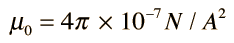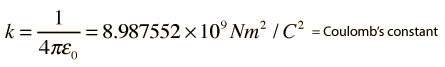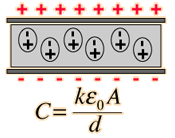# Electric Field

Electric field is defined as the electric force per unit charge. The direction of the field is taken to be the direction of the force it would exert on a positive test charge. The electric field is radially outward from a positive charge and radially in toward a negative point charge.Click on any of the examples above for more detail.Lorentz force law
 Using Gauss' law for electric field calculation
Index

Electric field concepts

Electromagnetic force

 HyperPhysics***** Electricity and Magnetism R Nave
Go Back

# Electric Field of Point Charge

 The electric field of a point charge can be obtained from Coulomb's law:The electric field is radially outward from the point charge in all directions. The circles represent spherical equipotential surfaces.The electric field from any number of point charges can be obtained from a vector sum of the individual fields. A positive number is taken to be an outward field; the field of a negative charge is toward it.

This electric field expression can also be obtained by applying Gauss' law.

 Other electric field geometries Multiple point charges
Index

Electric field concepts

 HyperPhysics***** Electricity and Magnetism R Nave
Go Back

# Electric and Magnetic Constants

In the equations describing electric and magnetic fields and their propagation, three constants are normally used. One is the speed of light c, and the other two are the electric permittivity of free space ε0 and the magnetic permeability of free space, μ0. The magnetic permeability of free space is taken to have the exact valueSee also relative permeability

This contains the force unit N for Newton and the unit A is the Ampere, the unit of electric current.

With the magnetic permeability established, the electric permittivity takes the value given by the relationshipwhere the speed of light c is given byThis gives a value of free space permittivitywhich in practice is often used in the formThese expressions contain the units F for Farad, the unit of capacitance, and C for Coulomb, the unit of electric charge.

In the presence of polarizable or magnetic media, the effective constants will have different values. In the case of a polarizable medium, called a dielectric, the comparison is stated as a relative permittivity or a dielectric constant. In the case of magnetic media, the relative permeability may be stated.

 Physical connections to permittivity and permeability
Index

Electric field concepts

 HyperPhysics***** Electricity and Magnetism R Nave
Go Back

# Physical Connections to Electric Permittivity and Magnetic Permeability

Expressions for the electric and magnetic fields in free space contain the electric permittivity ε0 and magnetic permeability μ0 of free space. As indicated in the section on electric and magnetic constants, these two quantities are not independent but are related to "c", the speed of light and other electromagnetic waves.The electric permittivity is connected to the energy stored in an electric field. It is involved in the expression for capacitance because it affects the amount of charge which must be placed on a capacitor to achieve a certain net electric field. In the presence of a polarizable medium, it takes more charge to achieve a given net electric field and the effect of the medium is often stated in terms of a relative permittivity.The magnetic permeability is connected to the energy stored in a magnetic field. It is involved in the expression for inductance because in the presence of a magnetizable medium, a larger amount of energy will be stored in the magnetic field for a given current through the coil. The effect of the medium is often stated in terms of a relative permeability.
Index

Electric field concepts

 HyperPhysics***** Electricity and Magnetism R Nave
Go Back